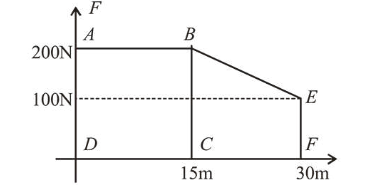# A person pushes a box on a rough horizontal platform surface.`
Question:

A person pushes a box on a rough horizontal platform surface. He applies a force of $200 \mathrm{~N}$ over a distance of $15 \mathrm{~m}$. Thereafter, he gets progressively tired and his applied force reduces linearly with distance to $100 \mathrm{~N}$. The total distance through which the box has been moved is $30 \mathrm{~m}$. What is the work done by the person during the total movement of the hox?

1. $3280 \mathrm{~J}$

2. $2780 \mathrm{~J}$

3. $5690 \mathrm{~J}$

4. $5250 \mathrm{~J}$

Correct Option: , 4

Solution:

(4) The given situation can be drawn graphically as shown in figure.

Work done $=$ Area under $F-x$ graph

$=$ Area of rectangle $A B C D+$ Area of trapezium $B C F E$$W=(200 \times 15)+\frac{1}{2}(100+200) \times 15=3000+2250$

$\Rightarrow W=5250 \mathrm{~J}$